# 2. Suppose a frm, tandard Ol, owns al t h land that can supportoll production, andis thus has a m...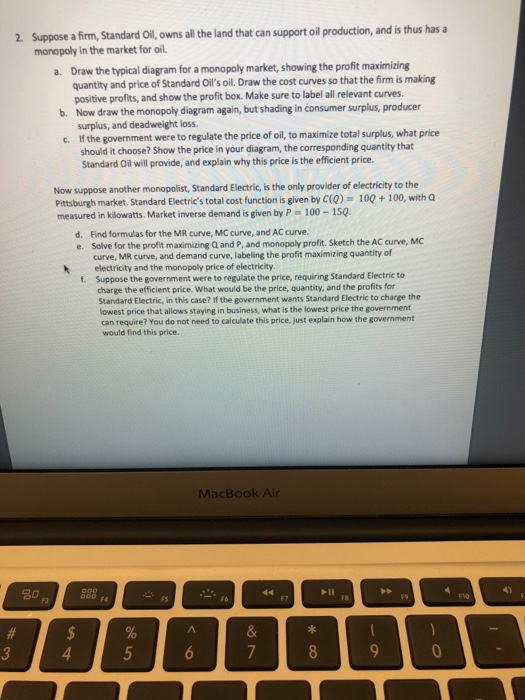2. Suppose a frm, tandard Ol, owns al t h land that can supportoll production, andis thus has a monopoly in the market for oil. a. Draw the typical diagram for a monopoly market, showing the profit maximizing quantity and price of Standard Oil'soil. Draw the cost curves so that the firm is making positive profits, and show the profit box. Make sure to label all relevant curves. Now draw the monopoly diagram again, but shading in consumer surplus, producer surplus, and deadweight loss If the government were to regulate the price of oil, to maximize total surplus, what price b. c. should it choose? Show the price in your diagram, the corresponding quantity that Standard Oil will provide, and explain why this price is the efficient price. Now suppose another monopolist, Standard Electric, is the only provider of electricity to the Pittsburgh market. Standard Electric's total cost function is given by C(Q) 10Q +100, with Q measured in kilowatts. Market inverse demand is given by P 100-150 Find formulas for the MR curve, MC curve, and AC curve. Solve for the profit maximizing Q and P, and monopoly profit. Sketch the AC curve, MC curve, MR curve, and demand curve, labeling the profit maximizing quantity of d. e. kelectricity and the monopoly price of electricity . Suppose the government were to regulate the price, requiring Standard Electric to charge the efficient price. What would be the price, quantity, and the profits for Standard Electric, in this case? If the government wants Standard Electric to charge the owest price that allows staying in business, what is the lowest price the government can require? You do not need to calculate this price, just explain how the government would find this price MacBook Air P11 FS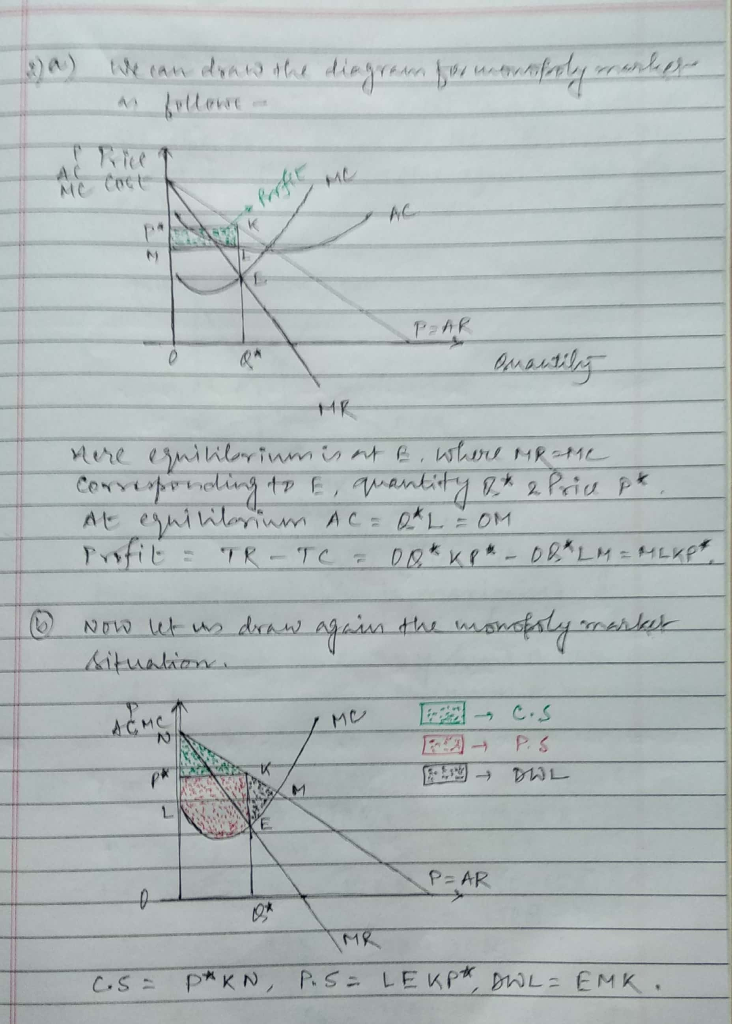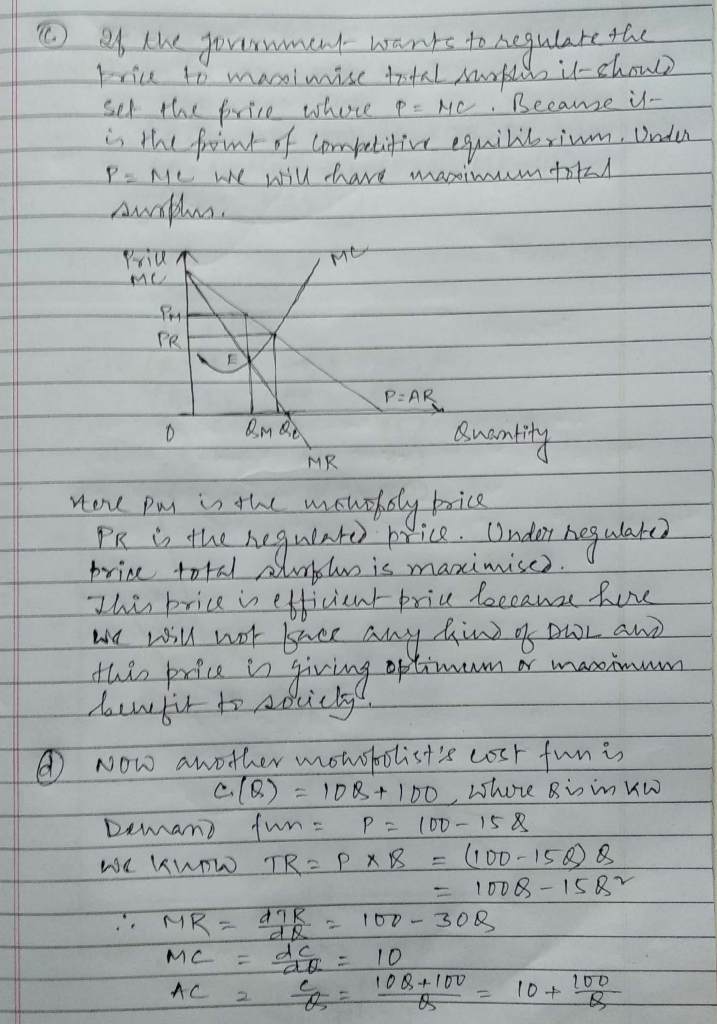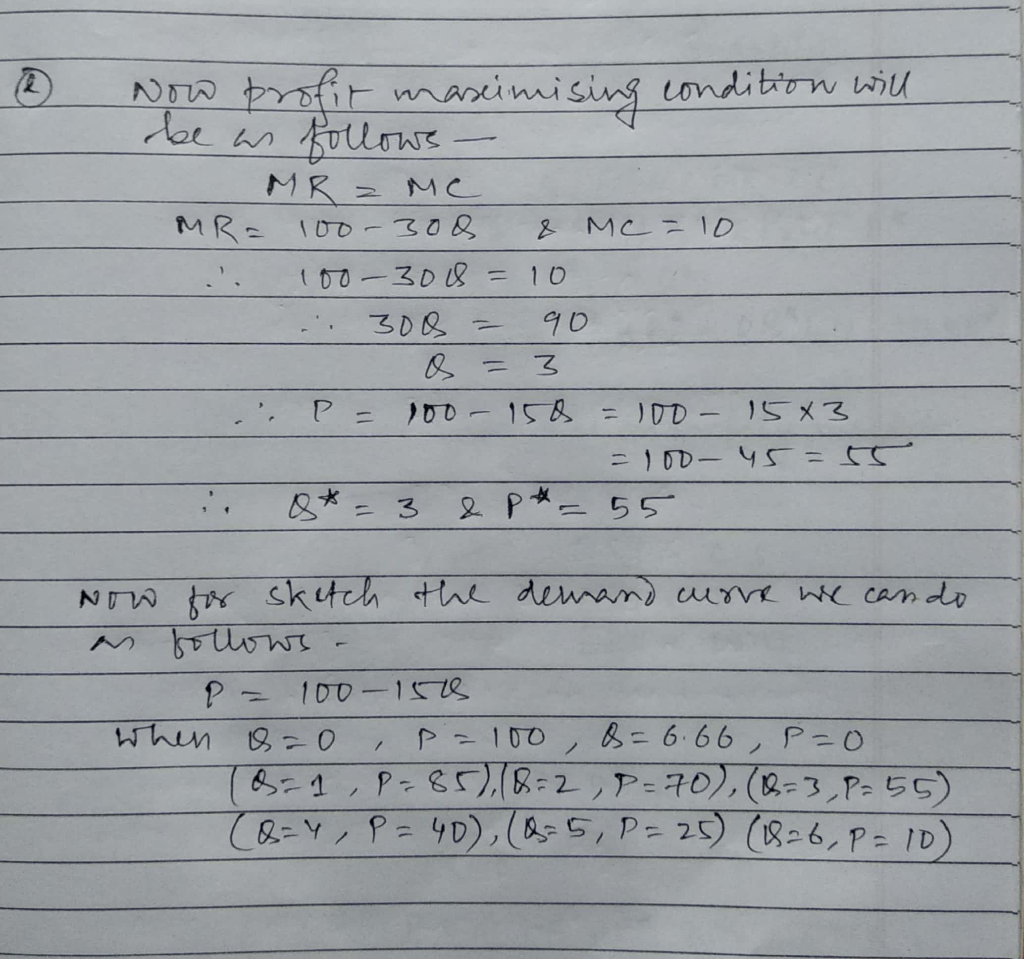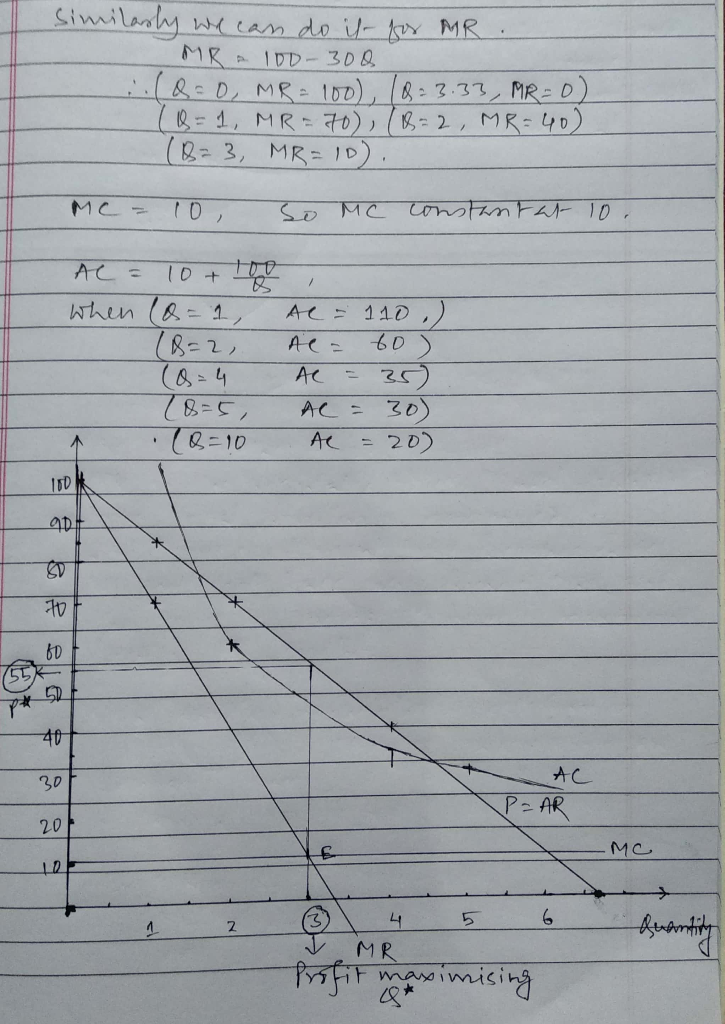##### Add Answer of: 2. Suppose a frm, tandard Ol, owns al t h land that can supportoll production, andis thus has a m...
More Homework Help Questions Additional questions in this topic.

• #### Let U = {x/x is a female} A = {x/x is a mathematician} B = {x/x owns a pickup} C = {x/x owns a dog} Describe in words a member of each of the following:

Need Online Homework Help?